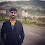### Spiral Order Matrix II - The Coding Shala

Home >> Interview Questions >> Spiral Order matrix 2

## Spiral Order Matrix II Problem Solution

Given an integer n, generate a square matrix filled with elements from 1 to n2 in spiral order.
Example:
Given n = 3,
You should return the following matrix:
``````[
[ 1, 2, 3 ],
[ 8, 9, 4 ],
[ 7, 6, 5 ]
]``````

#### Solution: (Java)

The concept is the same as how to print the Spiral Order Matrix.

Approach:
Let's see for n= 4, then numbers will come from 1 to 16(n*n) in the matrix.
As we need to insert numbers in spiral order so here we need 4 things to check, first and last column and top and bottom row. The following four steps we need to do:
1. First, we need to insert numbers in the top row. Our loop will go from the left column to the right then will increase the top row by one and now the top is our second row.
2. Now we are inserting the numbers in the right column, will start the loop from top to bottom, and then decrease the value of the right column by one. Now our right column is n-1 and so on later steps.
3. Now insert the numbers in the bottom row. the loop will go from right to left and then decrease the value of the bottom. the bottom will go up.
4. The last step is to insert the number in the left column. Loop will go from bottom to top row and then increase the value of the left column by one.
Once we follow all the above steps(one time) our outer layer is done all the left, right, top, bottom value updated. Follow these steps until left<=right and top<=bottom(outer loop).

Java Program:

 ``` 1 2 3 4 5 6 7 8 9 10 11 12 13 14 15 16 17 18 19 20 21 22 23 24 25 26 27 28 29``` ```public class Solution { public ArrayList> generateMatrix(int A) { int[][] res = new int[A][A]; int left=0,right=A-1,top=0,bottom=A-1,num=1;`````` //outer condition while(left<=right && top<=bottom){`````` //for top row for(int i=left;i<=right;i++) res[top][i] = num++; top++;`````` //for right column for(int i=top;i<=bottom;i++) res[i][right] = num++; right--;`````` // for bottom row for(int i=right;i>=left;i--) res[bottom][i] = num++; bottom--;`````` // for left column for(int i=bottom;i>=top;i--) res[i][left] = num++; left++; }`````` // insert data from 2-d array to arraylist ArrayList> ans = new ArrayList>(); for(int i=0;i tmp = new ArrayList(); for(int j=0;j

Other Posts You May Like

1.Could you explain the code with example please

1.